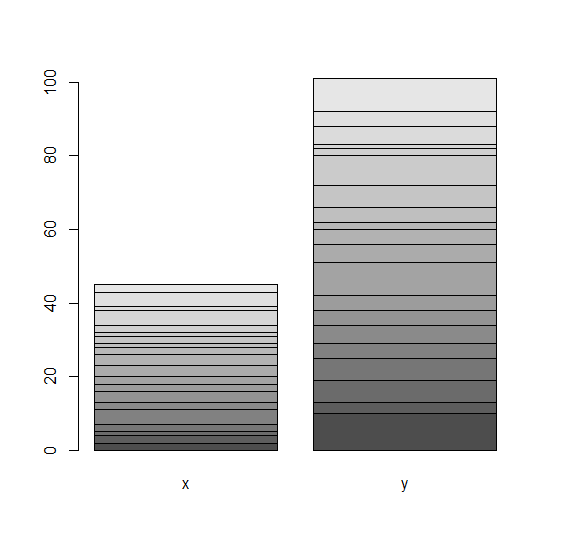# How to deal with error 'height' must be a vector or a matrix while creating barplot?

The error 'height' must be a vector or a matrix while creating barplot occurs when we provide data frame name instead of column names or read it with as.matrix. If we want to create bar plot for columns in a data frame then the data frame needs to be read as matrix.

For Example, if we have a data frame called df then we can create the barplot of columns in df by using the command given below −

barplot(as.matrix(df))

## Example

Following snippet creates a sample data frame −

df<-data.frame(x=rpois(20,2),y=rpois(20,5))
df

The following dataframe is created

    x y
1  2 10
2  2 3
3  1 6
4  2 6
5  4 4
6  2 5
7  3 4
8  2 4
9  2 9
10 3 5
11 3 4
12 2 2
13 1 4
14 2 6
15 1 8
16 2 2
17 4 1
18 1 5
19 4 4
20 2 9

To create the bar plot with data frame name on the above created data frame, add the following code to the above snippet −

df<-data.frame(x=rpois(20,2),y=rpois(20,5))
barplot(df)
Error in barplot.default(df) : 'height' must be a vector or a matrix

The correct way to create the bar plot is as follows −

df<-data.frame(x=rpois(20,2),y=rpois(20,5))
barplot(as.matrix(df))

## Output

If you execute all the above given snippets as a single program, it generates the following Output −